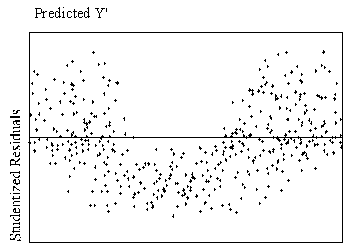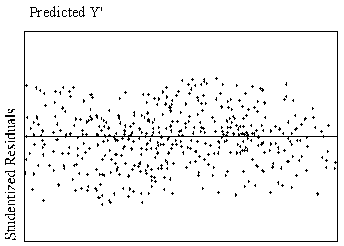# 迴歸分析的假設(Assumption of OLS regression)

Assumption 1：Linearity 線性(來源：http://pareonline.net/getvn.asp?v=8&n=2)

Assumption 2: Independence of the errors

Assumption 3: Homoscedasticity 同質性

Homoscedasticity，有人用homogeneity of variance，”Homoscedasticity means that the variance of errors is the same across all levels of the IV” (中文不大會翻譯，大意是說variance of errors在每個independent variable是一樣的)。什麼是variance of errors呢？就是每一個觀察值到regression line的距離，而這個距離的差異在每個independent variable是相當的。要看圖才懂的話，可看這：http://davidmlane.com/hyperstat/A121947.html

Assumption 4: Normality of the error distribution 錯誤呈現常態分配

Reference
Osborne, Jason & Elaine Waters (2002). Four assumptions of multiple regression that researchers should always test. Practical Assessment, Research & Evaluation, 8(2). Retrieved November 11, 2009 from http://PAREonline.net/getvn.asp?v=8&n=2 .